## Introduction

Temperature-sensing is a vital part of many Arduino projects.

This guide will show you how to use the TMP36 temperature sensor with a 100% compatible Arduino development board, the Little Bird Uno R3 boardto read the surrounding temperature.

With temperature detection, you could create a solar-powered temperature sensor, a biofeedback device that uses body temperature data, or even a smart coaster that lets you know when your coffee or tea is safe to drink!

1. ## Step 1 A Word of Warning!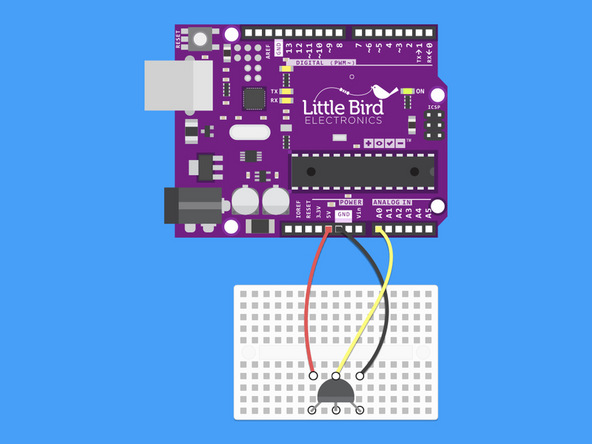• The TMP36 is a polarised part. The orientation of the TMP36 matters.

• If you don't hook it up correctly, it can get very very hot!

• In our examples, the flat edge (the bit with the writing) is pointed away from the Arduino.

2. ## Step 2 Insert the TMP36 Into the Breadboard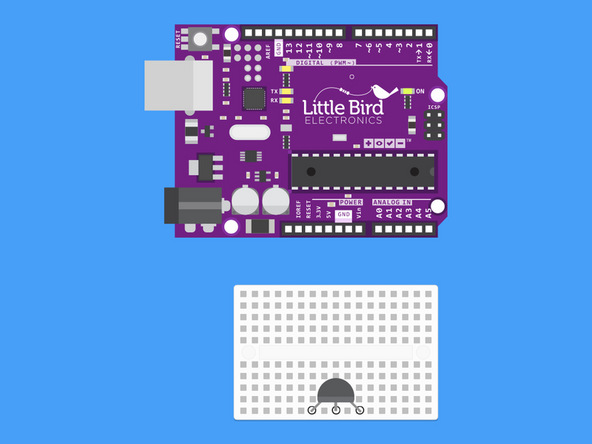• Insert the TMP36 Temperature Sensor into the breadboard so that the flat face of the sensor is facing away from the Arduino.

3. ## Step 3 Power supply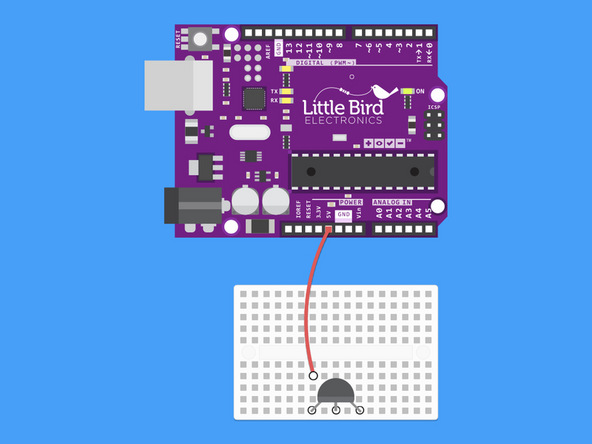• Connect the 5V line from the Arduino to the 5V pin of the TMP36.

4. ## Step 4 Voltage out pin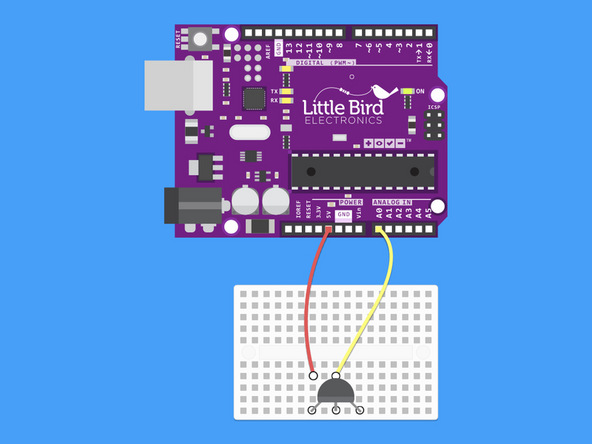• Connect Analogue Pin 0 to the TMP36's Voltage Out Pin (this is the middle pin).

5. ## Step 5 Ground pin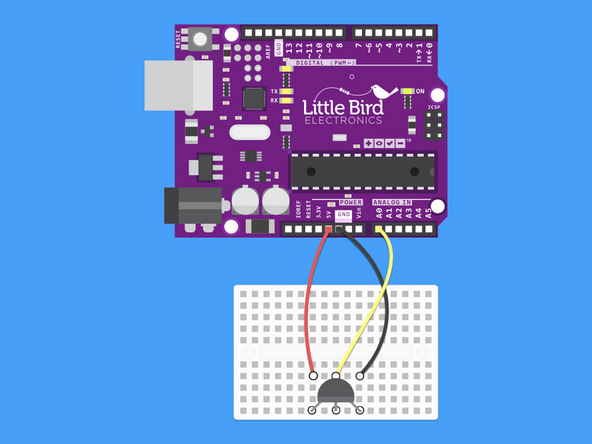• Connect the TMP36's ground pin to ground on the Arduino.

``````// We'll use analog input 0 to measure the temperature sensor's
// signal pin.

const int temperaturePin = A0;

void setup() {

Serial.begin(9600); //Initialize serial port & set baud rate to 9600 bits per second (bps)
}

void loop() {

float voltage, degreesC, degreesF; //Declare 3 floating point variables

voltage = getVoltage(temperaturePin); //Measure the voltage at the analog pin

degreesC = (voltage - 0.5) * 100.0; // Convert the voltage to degrees Celsius

degreesF = degreesC * (9.0 / 5.0) + 32.0; //Convert degrees Celsius to Fahrenheit

//Now print to the Serial monitor. Remember the baud must be 9600 on your monitor!
// These statements will print lines of data like this:
// "voltage: 0.73 deg C: 22.75 deg F: 72.96"

Serial.print("voltage: ");
Serial.print(voltage);
Serial.print("  deg C: ");
Serial.print(degreesC);
Serial.print("  deg F: ");
Serial.println(degreesF);

delay(1000); // repeat once per second (change as you wish!)
}

float getVoltage(int pin) //Function to read and return
//floating-point value (true voltage)
//on analog pin
{

// This equation converts the 0 to 1023 value that analogRead()
// returns, into a 0.0 to 5.0 value that is the true voltage
// being read at that pin.
}

// Other things to try with this code:

//   Turn on an LED if the temperature is above or below a value.

//   Read that threshold value from a potentiometer - now you've
//   created a thermostat!``````
• Copy this code and upload it to your Arduino using the Arduino IDE.

• View the temperature readings by opening the Serial monitor in your Arduino IDE.